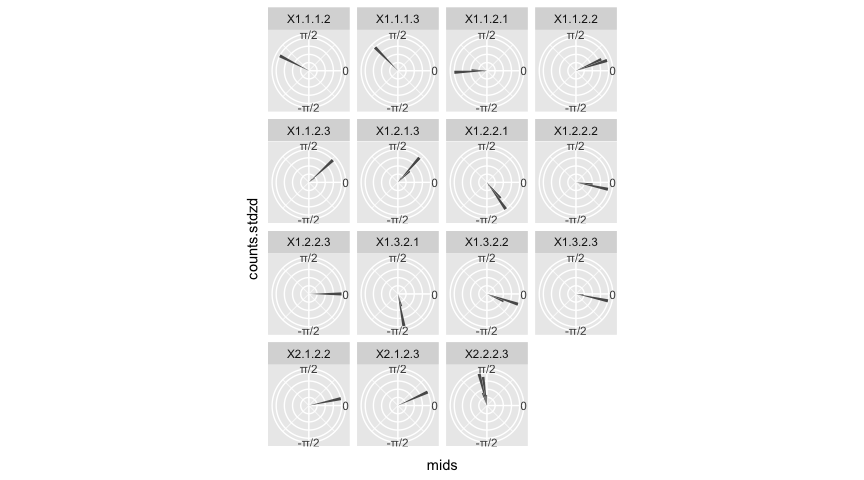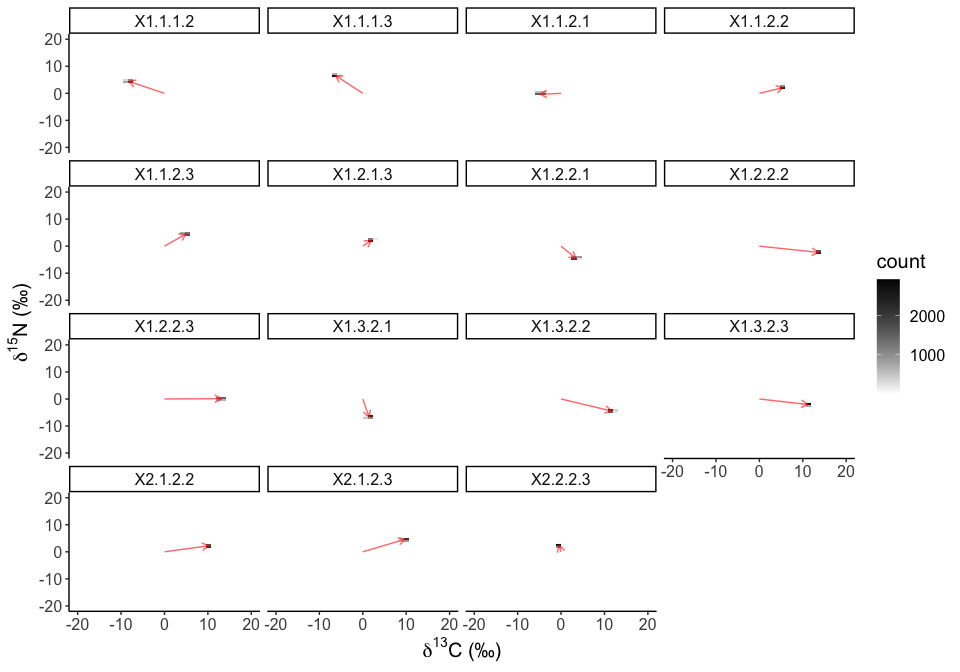# Vector Statistics on Centroids

#### 2023-08-22

## Loading required package: viridisLite

## Load up some data to illustrate the vector-based metrics

We will use the same demo data as per the main introductory vignette. We plot it just to remind us.

# remove previously loaded items from the current environment and remove previous graphics.
rm(list=ls())
graphics.off()

# Here, I set the seed each time so that the results are comparable.
# This is useful as it means that anyone that runs your code, *should*
# get the same results as you, although random number generators change
# from time to time.
set.seed(1)

library(SIBER)
library(ggplot2)
library(magrittr) # to enable piping
library(dplyr)
#>
#> Attaching package: 'dplyr'
#> The following objects are masked from 'package:stats':
#>
#>     filter, lag
#> The following objects are masked from 'package:base':
#>
#>     intersect, setdiff, setequal, union

# load in the included demonstration dataset
data("demo.siber.data")
#
# create the siber object
siber.example <- createSiberObject(demo.siber.data)

# Create lists of plotting arguments to be passed onwards to each
# of the three plotting functions.
community.hulls.args <- list(col = 1, lty = 1, lwd = 1)
group.ellipses.args  <- list(n = 100, p.interval = 0.95, lty = 1, lwd = 2)
group.hull.args      <- list(lty = 2, col = "grey20")

par(mfrow=c(1,1))
plotSiberObject(siber.example,
hulls = F, community.hulls.args,
ellipses = T, group.ellipses.args,
group.hulls = T, group.hull.args,
bty = "L",
iso.order = c(1,2),
xlab = expression({delta}^13*C~'\u2030'),
ylab = expression({delta}^15*N~'\u2030')
)
#> Warning in title(...): font metrics unknown for Unicode character U+2030

#> Warning in title(...): font metrics unknown for Unicode character U+2030
#> Warning in title(...): conversion failure on '‰' in 'mbcsToSbcs': dot
#> substituted for <e2>
#> Warning in title(...): conversion failure on '‰' in 'mbcsToSbcs': dot
#> substituted for <80>
#> Warning in title(...): conversion failure on '‰' in 'mbcsToSbcs': dot
#> substituted for <b0>
#> Warning in title(...): font metrics unknown for Unicode character U+2030

#> Warning in title(...): font metrics unknown for Unicode character U+2030
#> Warning in title(...): conversion failure on '‰' in 'mbcsToSbcs': dot
#> substituted for <e2>
#> Warning in title(...): conversion failure on '‰' in 'mbcsToSbcs': dot
#> substituted for <80>
#> Warning in title(...): conversion failure on '‰' in 'mbcsToSbcs': dot
#> substituted for <b0>

As before, we fit the Bayesian model describing the ellipses.


# options for running jags
parms <- list()
parms$n.iter <- 2 * 10^4 # number of iterations to run the model for parms$n.burnin <- 1 * 10^3 # discard the first set of values
parms$n.thin <- 10 # thin the posterior by this many parms$n.chains <- 2        # run this many chains

parms$save.output = FALSE parms$save.dir = tempdir()

# define the priors
priors <- list()
priors$R <- 1 * diag(2) priors$k <- 2
priors$tau.mu <- 1.0E-3 # fit the ellipses which uses an Inverse Wishart prior # on the covariance matrix Sigma, and a vague normal prior on the # means. Fitting is via the JAGS method. ellipses.posterior <- siberMVN(siber.example, parms, priors) #> Compiling model graph #> Resolving undeclared variables #> Allocating nodes #> Graph information: #> Observed stochastic nodes: 30 #> Unobserved stochastic nodes: 3 #> Total graph size: 45 #> #> Initializing model #> #> Compiling model graph #> Resolving undeclared variables #> Allocating nodes #> Graph information: #> Observed stochastic nodes: 30 #> Unobserved stochastic nodes: 3 #> Total graph size: 45 #> #> Initializing model #> #> Compiling model graph #> Resolving undeclared variables #> Allocating nodes #> Graph information: #> Observed stochastic nodes: 30 #> Unobserved stochastic nodes: 3 #> Total graph size: 45 #> #> Initializing model #> #> Compiling model graph #> Resolving undeclared variables #> Allocating nodes #> Graph information: #> Observed stochastic nodes: 30 #> Unobserved stochastic nodes: 3 #> Total graph size: 45 #> #> Initializing model #> #> Compiling model graph #> Resolving undeclared variables #> Allocating nodes #> Graph information: #> Observed stochastic nodes: 30 #> Unobserved stochastic nodes: 3 #> Total graph size: 45 #> #> Initializing model #> #> Compiling model graph #> Resolving undeclared variables #> Allocating nodes #> Graph information: #> Observed stochastic nodes: 30 #> Unobserved stochastic nodes: 3 #> Total graph size: 45 #> #> Initializing model ## Introducing the vector-based statistics. Now we can extract the centroid data and plot the vector data. # extract the centroids from the fitted model object centroids <- siberCentroids(ellipses.posterior) # calculate pairwise polar vectors among all groups # this is not actually used in this example angles_distances <- allCentroidVectors(centroids, do.plot = FALSE) The posterior distributions of the angles can then be visualise as a polar histograms, since the data wrap between $$-\pi$$ and $$\pi$$. For some reason, I cant get the gridline associate with $$\pi$$ to appear.  # = = = = = = = = = = = = = = = = = = = = = = = = = = = = = = = = = = = = = = = = # function to do the histograms on each group my.hist <- function(df){ test <- hist(df$angles,
breaks = seq(from = -pi, to = pi, length = 60),
plot = FALSE)

X <- data.frame(counts = test$counts, mids = test$mids, dens = test$density, counts.stdzd = test$counts / max(test$counts)) return(X) } # = = = = = = = = = = = = = = = = = = = = = = = = = = = = = = = = = = = = = = = = # calculate the points for each group's ellipse hist.by.groups <- angles_distances %>% group_by(comparison) %>% do(my.hist(.)) all.roses <- ggplot(data = hist.by.groups, aes(x = mids, y = counts.stdzd)) + geom_bar(stat = "identity") + coord_polar(start = pi / 2, direction = -1) + facet_wrap( ~ comparison) + theme(axis.ticks.y = element_blank(), axis.text.y = element_blank()) + scale_x_continuous( breaks = c(-pi, -pi/2, 0, pi/2, pi), labels = c("","-\u03C0/2","0","\u03C0/2", "\u03C0")) print(all.roses)## Polar density plots Try some fancy 2-d density plots. These boxes represent the density of the tips of the polar vectors describing the relative position between pairs of individuals. The median vector is shown as an arrow, with the 2-dimensional heatmaps showing where the tip is likely to be. To do this, we have to convert our polar coordinates to cartesian space using the formulae: $$(x,y) = (r\cos(\theta),r\sin(\theta))$$. The names for each comparison are not the prettiest or most informative. Here the “X” is superfluous and has appeared via the dplyr code above. The panel label ‘X1.1.1.2’ reads as community 1 group 1, compared with community 1 group 2. You could relabel the groups in the field cart_positions$comparison below.


median_vectors <- dplyr::summarise(group_by(angles_distances, comparison),
medAngle = median(angles), medDist = median(distances))

origins <- data.frame(comparison = median_vectors$comparison, x = 0, y = 0) ends <- with(median_vectors, data.frame(comparison = comparison, x = medDist * cos(medAngle), y = medDist * sin(medAngle))) # = = = = = = = = = = = = = = = = = = = = = = = = = = = = = = = = = = = = = = = = # generate the start and end points of the medians for the arrows for_arrows <- dplyr::bind_rows(origins, ends) # rename the comparison label for nice plot labels below # aa <- unlist(strsplit(as.character(for_arrows$comparison), "[.]"))
# aa <- aa[seq(3,length(aa),5)]
# for_arrows$comparison2 <- factor(aa) # = = = = = = = = = = = = = = = = = = = = = = = = = = = = = = = = = = = = = = = = # create the cartesian points for the estimated tips of the arrows cart_positions <- with(angles_distances, data.frame(x = distances * cos(angles), y = distances * sin(angles), comparison = comparison )) # rename as above # bb <- unlist(strsplit(as.character(angles_distances$comparison), "[.]"))
# bb <- bb[seq(3,length(bb),5)]
# cart_positions\$comparison2 <- factor(bb)

# = = = = = = = = = = = = = = = = = = = = = = = = = = = = = = = = = = = = = = = =
# plot it
ggplot(cart_positions, aes(x,y) ) +
geom_bin2d(bins = 20) +
scale_fill_gradient(low = "white", high = "black") +
coord_cartesian(xlim = c(-20, +20), ylim = c(-20, +20)) +
facet_wrap( ~ comparison, scales = "fixed") +
theme_classic() +
geom_path(data = for_arrows,
arrow = arrow(type = "open", length = unit(0.2, "cm")),
col = "red", alpha = 0.6) +
ylab(expression(paste(delta^{15}, "N (\u2030)"))) +
xlab(expression(paste(delta^{13}, "C (\u2030)"))) +
theme(text = element_text(size=15))